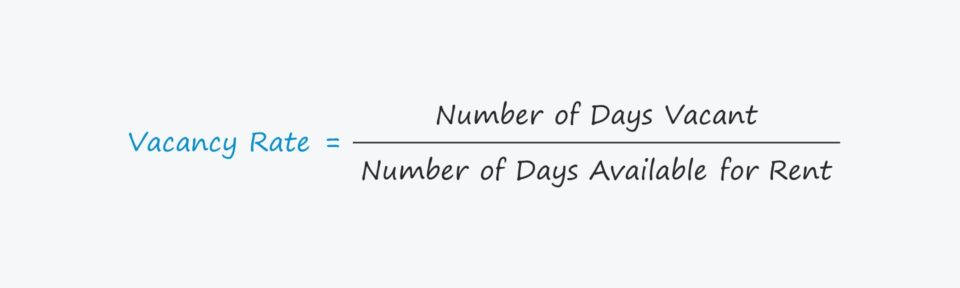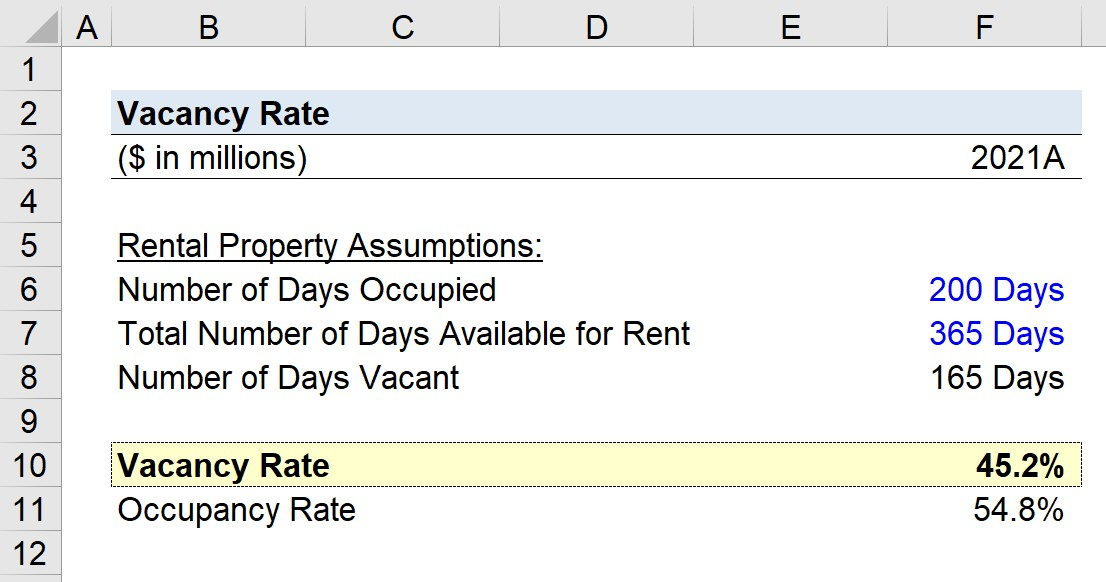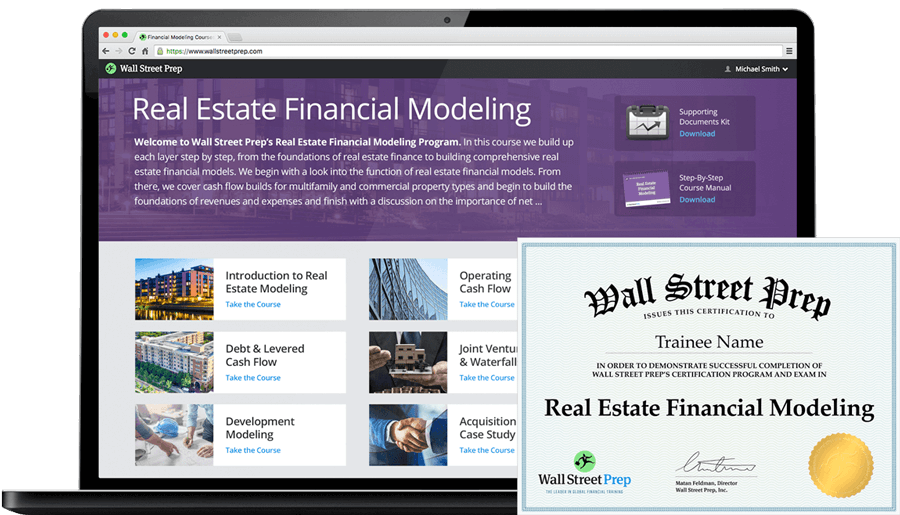# Vacancy Rate

Guide to Understanding the Vacancy Rate## How to Calculate the Vacancy Rate

The vacancy rate measures the proportion of rental units that are unoccupied at a particular time and quantifies the dollar amount of rental income lost from unoccupied units throughout a certain time frame.

The rate of vacancy is a key driver of revenue in the following industries:

• Hospitality Industry (Hotels)
• Apartment Complex
• Healthcare Industry (Hospitals, Assisted Living Facilities)
• Rental Platforms (Airbnb)

Because vacancy is directly tied to rental revenue, the metric can be utilized to assess historical performance and market behavior (i.e. seasonality, cyclicality), as well as forecast future demand.

Backward-looking historical data collected by a property manager or real estate investor can be used to help determine pricing and marketing strategies going forward.

## Vacancy Rate Formula

The formula for calculating the vacancy rate on a rental property is as follows.

###### Formula
• Vacancy Rate = Number of Days Vacant ÷ Total Number of Days Available for Rent

For example, if a single-family rental available for 365 days in a year was vacant for two months out of the twelve-month period, the rate of vacancy is 16.4% (60 Days ÷ 365 Days)

## Vacancy Rate vs. Occupancy Rate

The vacancy rate is the inverse of the occupancy rate, so the formulas for calculating the occupancy rate on a particular date and on an annual basis are as follows.

###### Formula
• Occupancy Rate, Single Date = Number of Occupied Rental Units ÷ Total Number of Available Rental Units
• Occupancy Rate, Annual = Number of Days Occupied ÷ Total Number of Available for Rent

Moreover, the formula below is an alternative method to calculate the vacancy rate using the occupancy rate.

###### Formula
• Vacancy Rate = 1 – Occupancy Rate

## How to Interpret the Vacancy Rate

In order to optimize operating efficiency and maximize rental income, properties should attempt to reduce their rate of vacancy over time, all else being equal.

• Low Vacancy → Higher Rental Income
• Higher Vacancy → Lower Rental Income

While a higher occupancy rate is perceived as a positive sign when the underlying driver is related to increased demand from consumers, which allows the property owner to raise prices and become more profitable.

If the occupancy rate is increased by undercutting competitors’ prices, the effect on revenue and profits could actually be negative.

For property owners with a diverse portfolio of rental properties, vacancy is an important consideration when comparing performance between properties.

Understanding if the demand among renters is rising around a certain location can enable the property owner to set prices appropriately to pocket more profits and capitalize on these trends.

In contrast, becoming aware that renters are shifting away from an area is typically a red flag and can lead to convincing an owner to sell the property before it loses more value.

## Vacancy Rate Calculator – Excel Template

We’ll now move to a modeling exercise, which you can access by filling out the form below.Submitting ...

## Airbnb Vacancy Rate Example Calculation

Suppose an Airbnb host is attempting to calculate the vacancy rate of their rental property.

In 2021, the rental property was listed as available for rent each day of the entire year.

Of the 365 days that the property was available, the number of days the room was occupied was 200 days.

• Number of Days Occupied = 200 Days
• Total Number of Days Available for Rent = 365 Days

Given those two assumptions, we can calculate the number of days that the room was unoccupied as 165 days.

• Number of Days Vacant = 365 Days – 200 Days = 165 Days

By dividing the number of vacant days by the total number of days available for rent, we arrive at 45.2%.

• Vacancy Rate = 165 Days ÷ 365 Days = 45.2%

From there, we can also back-solve the occupancy rate as 54.8% by subtracting the vacancy rate from one.

• Occupancy Rate = 1 – 45.2% = 54.8%20+ Hours of Online Video Training

#### Master Real Estate Financial Modeling

This program breaks down everything you need to build and interpret real estate finance models. Used at the world's leading real estate private equity firms and academic institutions.